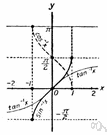# inverse function

Also found in: Thesaurus, Encyclopedia, Wikipedia.

## inverse function

n. Mathematics
A function whose relation to a given function is such that their composite is the identity function. It is often found by interchanging dependent and independent variables.

## in′verse func′tion

n.
Math. the function that replaces another function when the dependent and independent variables of the first function are interchanged for an appropriate set of values of the dependent variable.
[1810–20]
ThesaurusAntonymsRelated WordsSynonymsLegend:
 Noun 1inverse function - a function obtained by expressing the dependent variable of one function as the independent variable of another; f and g are inverse functions if f(x)=y and g(y)=xfunction, mapping, mathematical function, single-valued function, map - (mathematics) a mathematical relation such that each element of a given set (the domain of the function) is associated with an element of another set (the range of the function)
Based on WordNet 3.0, Farlex clipart collection. © 2003-2012 Princeton University, Farlex Inc.
References in periodicals archive ?
Daniel Patrick Moynihan who said: 'The amount of violations of human rights is always an inverse function of the amount of complaints about human rights violations heard from there.
The inverse distance weighting or inverse distance weighted (IDW) method evaluate the values of an attribute at un-sampled points using a linear addition of values at sampled points weighted by an inverse function of the distance from the point of interest to the sampled points :
By approximating the inverse function of free boundary, the free-boundary PDE could be written as a unified form of PDE defined on a fixed space region; then applying Laplace transform to the unified PDE with respect to time variable results in a boundary value problem of ODE; finally the finite difference method (FDM) is adopted for solving the aimed ODE, and the computational results are restored via the numerical Laplace inversion.
When asked whether or not a function has an inverse function on a particular interval, many students automatically turn to the horizontal line test.
One way of finding such a third sub-step iteration with a feasible error corrector is to apply the Inverse Function Theorem  to (2.3).
Now the TELM defines the matrix [W.sub.HE] = [[B.sub.1] [W.sub.1]], so the parameters of the second hidden layer can be easily obtained by using formula (12) and the inverse function of the activation function.
Let the function f given by (1.1) be in the class H(q) and [f.sup.-1](w) near w = 0 is the inverse function of f, then
The receiver knows the equation that the sender has used to disguise the wave and can therefore use the inverse function to cast off that disguise.
For each level antidepressant dosage, the depression score was an inverse function of rising time.
Indeed, the inverse function may have an analytic continuation to A, with
In order to find this reparametrization t([theta]), [theta] [member of] [0,2[pi]], we first construct the inverse function [theta](t) = Arg([zeta](z))[|.sub.z=z(t)[member of]L].

Site: Follow: Share:
Open / Close